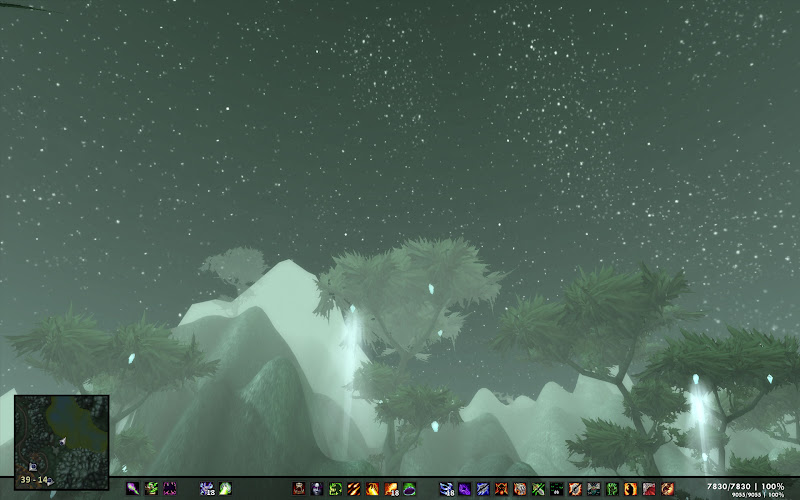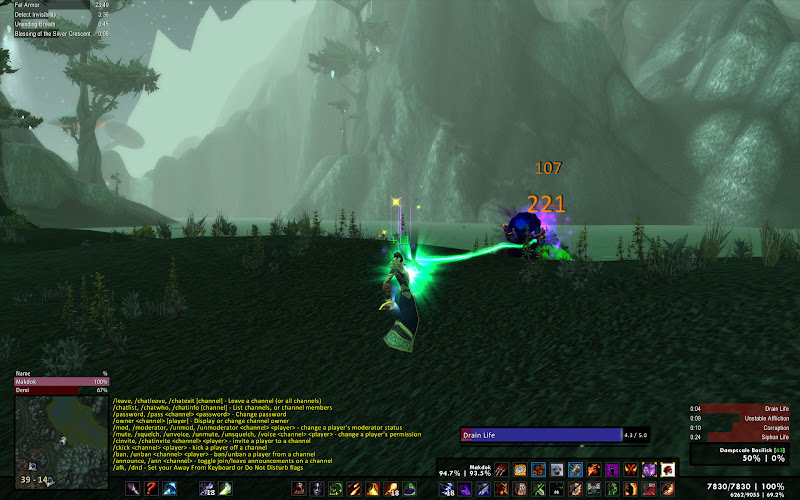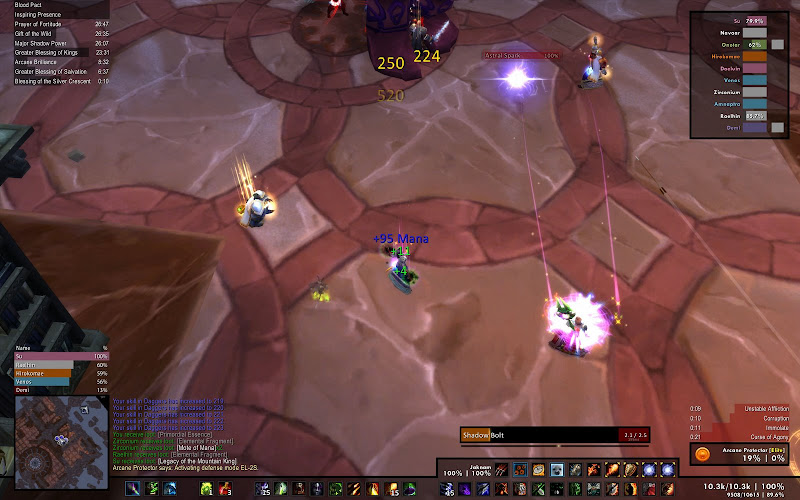• 0

posted a message on kgPanels Offical Thread
Man, I've been working all weekend and I'm stomped.

Parenting to bars or unit frames isn't an option, so I need to use scripts to show and hide frames based on whether I have a pet out or a target.

I set up a frame (and a number of extras parented to it) to show when I have a pet and hide when I do not. I've used code that I've used to do this in the past, but now it seems buggy. It'll work, but then if I add more frames, even without scripts on them, it'll just stop working.

```self:RegisterEvent("UNIT_PET")
self:Hide()```
OnEvent
```if UnitExists("pet") == nil then
self:Hide()
else
self:Show()
end```
Also... I need to show/hide a frame to fill in a gap when another frame is not present. It appears the best way to do that would be to use "kgPanel#:Show()" in the OnHide script area, and the opposite in the OnShow one, of the other frame... but it seems the # for the frame changes each time I log in. It was 7, then 4, and then 5. Is there a static method to refer to direct a frame from within the script of another? I don't recall these numbers being random in the past.
• 0

posted a message on For you Warlocks, what addons do you use specific to you?
I have my UI in the post your UI thread. The only lock-specific addon I use is EEshards, so I don't have to manually delete shards. Otherwise I find that most of the functionality I would want for my lock is about the same as I would want for most dps classes.
Posted in: General Chat
• 0

posted a message on kgPanels Offical Thread
Quote from Kagaro »

I put in a fix for that last night, as someone else was having the problem. What did you parent the frame to?

Parent is UIParent. Anchor is "Bottom" (one of my other panels).

<edit: so I reparented to "Bottom" after posting this and it seems to work okay. I'm good with that.>
• 0

posted a message on UI Screenshots - Show us what you use!
Very impressive work Hildy. It's kind of similar to the setup I posted a while back but with far better execution lol.

Thanks! If you've posted it here, on the official forums, or at elitist jerks it is entirely possible it has made it's way into the bottomless folder of inspirational UI pics that I mine when I need ideas. :)

Hildy, I cant understand how you can live with Unit Frames in such a strange place, let alone its just text and not a bar to easily Identify.

The text was a key component in my original design. I don't seem to have an issue tracking the numbers on a few unit frames, but I went with bars on party/raid members for exactly the reason you state. I was afraid I wouldn't react as quickly as I would to a visual bar when having to scan so many units. Note that my own unit frame and pet show up in the party/raid frame both when I am in a raid or in a group, so I get the visual for my health when not solo as well. When solo the numbers aren't really that hard to track.

Most everything I need to see in combat, on a dps character, is centered on the bottom right corner (which I have found is where my eye and mouse pointer tend to settle on the screen). My core spell rotations (DoTs, Shadowbolt, and everything needed to fear juggle) are keybound... so I only go to the buttons with my mouse for the odd buff, banish, or summon.

I've considered a healer variant (Symbiont0.3h) which trades the positions of the target and party/raid frame so that I could get those health bars down into my sweet spot to be used with a click casting addon.
Posted in: General Chat
• 0

posted a message on kgPanels Offical Thread
I'm having a *different* issue now. I have two frames that hide or show when my pet is out. The one that hides when my pet isn't out and shows when he is, is working just fine. The other one is not.

Here is what I have...

```self:RegisterEvent("UNIT_PET")
self:Hide()```

OnEvent
```if UnitExists("pet") then
self:Hide()
else
self:Show()
end```

This worked fine before running WAU this evening. Now the frame is visible anytime my UI reloads, whether my pet is out or not, and does not respond if I dismiss/resummon. The odd part is that if I go in and hit the "Apply" button under the code windows for OnLoad and OnEvent the frame immediately resets and works correctly until my UI reloads... and then it stops working again.
• 0

posted a message on UI Screenshots - Show us what you use!
At rest
The bottom bar is 30 buttons. Pressing alt pages them to a second page of 30 buttons. A hidden Fubar under the minimap is used to give access to data collection and general convenience addons.Solo Combat
The pet bar "attaches" to the bottom bar and includes the pet unit frame. Chat appears above the left side of the bar to a maximum height equal with the top of the minimap. The target unit frame appears in a box above the player unit frame, with buffs/debuffs on the target appearing above it. Buffs/debuffs on the player appear in the upper left corner. You can see Omen above the minimap (grows up from the minimap and expands to 6 bars) and the casting bar above the pet bar. A small casting bar appears beneath the target unit frame if the target casts.Group/Raid
The party/raid frame appears when I join a group. It doubles in size (as pictured) for a 10 man raid, and then increases an equal amount for 15, 20, and 25 person raids. The squares you see behind people's health bars are pet health. I used bars for health here as the text used everywhere else would be too much to process mentally in a fast paced raid environment. I may build a healer variant that swaps the target and group/raid positions to allow for easier access via a click-casting addon.Unseen
Tooltips and Aloft are skinned, sorta, to match the rest of the UI. OneBag is not. Neither are the various skill, spell, character, guild, and other panels. Those just aren't open frequently enough to worry about making them match.

I've performed a few minor cosmetic updates since these screenshots were taken.

Aloft, Bartender3, BigWigs, LittleWigs, ClearFont2, CowTip, CyCircled, CyCircled_VolM, eeShards, ElkBuffBars, ErrorMonster, FuBar, FuBar_ExperienceFu, FuBar_RecountFu, FuBar_TrackerFu, ItemPriceTooltip, kgPanels, Omen, OmniCC, OneBag, OneBank, OneRing, oRA2, Outfitter, PitBull, Quartz, QuestHelper, RatingBuster, Recount, SharedMedia, SimpleChatMods, SimpleMiniMap... and I'm always looking for the newest great toy.

* = use the magnifying glass in the upper right corner on the links to larger images to see the actual full size screen shots.
Posted in: General Chat
• 0

posted a message on kgPanels Offical Thread
I appreciate you looking into it. It still doesn't seem to be working for me. I'm sure it is probably something I'm doing. Luckily it isn't something my UI depends on. Otherwise these frames have been great to work with.
• 0

posted a message on kgPanels Offical Thread
Sorry to be a bother. It bugs me when I can't figure this sorta thing out.

`^1^T^SBottom~`Border~`Middle^T^Sstrata^SBACKGROUND^SanchorTo^STOPRIGHT^Sparent^SUIParent^Sbg_orientation^SHORIZONTAL^SanchorFrom^SBOTTOMRIGHT^Shflip^b^Sbg_insets^T^Sr^N4^St^N4^Sl^N-4^Sb^N-4^t^Svflip^b^StileSize^N0^Sbg_texture^SNone^Sanchor^SBottom^Slevel^N0^Sscripts^T^SEVENT^Sif~`UnitExists("pet")~`then~J~`~`~`~`~`self:Hide()~Jelse~J~`~`~`~`~`self:Show()~Jend^SLOAD^Sself:RegisterEvent("UNIT_PET")^t^Sbg_blend^SBLEND^Sborder_texture^SNone^Sbg_style^SSOLID^Sgradient_color^T^Sa^N1^Sb^N0^Sg^N0^Sr^N0^t^Sborder_color^T^Sa^N1^Sb^N0^Sg^N0^Sr^N0^t^Stext^T^Sy^N0^Sx^N0^SjustifyH^SCENTER^Sfont^SBlizzard^Scolor^T^Sa^N1^Sb^N1^Sg^N1^Sr^N1^t^Stext^S^SjustifyV^SMIDDLE^Ssize^N12^t^Sx^S-233^Swidth^S462^Srotation^N0^Sbg_color^T^Sa^N1^Sb^N0^Sg^N0^Sr^N0^t^Sbg_alpha^N1^Sborder_edgeSize^N16^Sheight^S12^Smouse^b^Sy^S-8^Stiling^b^t^STarget~`Border~`Right^T^Sstrata^SBACKGROUND^SanchorTo^SBOTTOMRIGHT^Sparent^STarget^Sbg_orientation^SHORIZONTAL^SanchorFrom^SBOTTOMLEFT^Shflip^b^Sbg_insets^T^Sb^N-4^St^N4^Sl^N-4^Sr^N4^t^Svflip^b^StileSize^N0^Sscripts^T^t^Sanchor^STarget^Slevel^N0^Sbg_texture^SNone^Sbg_blend^SBLEND^Sy^S0^Sbg_style^SSOLID^Sgradient_color^T^Sa^N1^Sr^N0^Sg^N0^Sb^N0^t^Sborder_color^T^Sa^N1^Sr^N0^Sg^N0^Sb^N0^t^Sborder_texture^SNone^Sbg_color^T^Sa^N1^Sr^N0^Sg^N0^Sb^N0^t^Swidth^S12^Srotation^N0^Sx^S-8^Sbg_alpha^N1^Sborder_edgeSize^N16^Sheight^S45^Smouse^b^Stext^T^Sy^N0^Sfont^SBlizzard^SjustifyH^SCENTER^Sx^N0^Scolor^T^Sa^N1^Sr^N1^Sg^N1^Sb^N1^t^Stext^S^SjustifyV^SMIDDLE^Ssize^N12^t^Stiling^b^t^SPet~`Border~`Left^T^Sstrata^SBACKGROUND^SanchorTo^SBOTTOMLEFT^Sparent^SPet^Sbg_orientation^SHORIZONTAL^SanchorFrom^SBOTTOMRIGHT^Shflip^b^Sbg_insets^T^Sr^N4^St^N4^Sl^N-4^Sb^N-4^t^Svflip^b^StileSize^N0^Sbg_texture^SNone^Sanchor^SPet^Slevel^N0^Sscripts^T^t^Sbg_blend^SBLEND^Sborder_texture^SNone^Sbg_style^SSOLID^Sgradient_color^T^Sa^N1^Sb^N0^Sg^N0^Sr^N0^t^Sborder_color^T^Sa^N1^Sb^N0^Sg^N0^Sr^N0^t^Stext^T^Sy^N0^Sx^N0^SjustifyH^SCENTER^Sfont^SBlizzard^Scolor^T^Sa^N1^Sb^N1^Sg^N1^Sr^N1^t^Stext^S^SjustifyV^SMIDDLE^Ssize^N12^t^Sx^S8^Swidth^S12^Srotation^N0^Sbg_color^T^Sa^N1^Sb^N0^Sg^N0^Sr^N0^t^Sbg_alpha^N1^Sborder_edgeSize^N16^Sheight^S43^Smouse^b^Sy^S0^Stiling^b^t^SMinimap^T^Sstrata^SBACKGROUND^SanchorTo^SCENTER^Sparent^SMinimap^Sbg_orientation^SHORIZONTAL^SanchorFrom^SCENTER^Shflip^b^Sbg_insets^T^Sr^N4^St^N4^Sl^N-4^Sb^N-4^t^Svflip^b^StileSize^N0^Sbg_texture^SNone^Sanchor^SMinimap^Slevel^N0^Sscripts^T^t^Sbg_blend^SBLEND^Sborder_texture^SNone^Sbg_style^SSOLID^Sgradient_color^T^Sa^N1^Sb^N1^Sg^N1^Sr^N1^t^Sborder_color^T^Sa^N1^Sb^N1^Sg^N1^Sr^N1^t^Stext^T^Sy^N0^Sx^N0^SjustifyH^SCENTER^Sfont^SBlizzard^Scolor^T^Sa^N1^Sb^N1^Sg^N1^Sr^N1^t^Stext^S^SjustifyV^SMIDDLE^Ssize^N12^t^Sx^N0^Swidth^S109.5%^Srotation^N0^Sbg_color^T^Sa^N1^Sb^N0^Sg^N0^Sr^N0^t^Sbg_alpha^N1^Sborder_edgeSize^N16^Sheight^S110%^Smouse^b^Sy^N0^Stiling^b^t^SCastBar^T^Sstrata^SBACKGROUND^SanchorTo^STOPRIGHT^Sparent^SQuartzCastBar^Sbg_orientation^SHORIZONTAL^SanchorFrom^SBOTTOMRIGHT^Shflip^b^Sbg_insets^T^Sr^N4^St^N4^Sl^N-4^Sb^N-4^t^Svflip^b^StileSize^N0^Sbg_texture^SNone^Sanchor^SBottom^Slevel^N0^Sscripts^T^SLOAD^Sself:SetHeight(40)~Jself:SetWidth(370)^t^Sbg_blend^SBLEND^Sborder_texture^SNone^Sbg_style^SSOLID^Sgradient_color^T^Sa^N1^Sb^N0^Sg^N0^Sr^N0^t^Sborder_color^T^Sa^N1^Sb^N0^Sg^N0^Sr^N0^t^Stext^T^Sy^N0^Sx^N0^SjustifyH^SCENTER^Sfont^SBlizzard^Scolor^T^Sa^N1^Sb^N1^Sg^N1^Sr^N1^t^Stext^S^SjustifyV^SMIDDLE^Ssize^N12^t^Sx^S-284^Swidth^S360^Srotation^N0^Sbg_color^T^Sa^N1^Sb^N0^Sg^N0^Sr^N0^t^Sbg_alpha^N1^Sborder_edgeSize^N16^Sheight^S41^Smouse^b^Sy^S58^Stiling^b^t^SParty^T^Sstrata^SBACKGROUND^SanchorTo^STOPRIGHT^Sparent^SUIParent^Sbg_orientation^SHORIZONTAL^SanchorFrom^STOPRIGHT^Shflip^b^Sbg_insets^T^Sb^N-4^St^N4^Sl^N-4^Sr^N4^t^Svflip^b^StileSize^N0^Sscripts^T^SEVENT^Slocal~`pmems~`=~`GetNumPartyMembers()~Jlocal~`rmems~`=~`GetNumRaidMembers()~J--~`~Jif~`(rmems~`<~`6)~`and~`(pmems~`>~`0)~J~`~`~`~`~`then~J~`~`~`~`~`self:Show()~J~`~`~`~`~`self:SetHeight(128)~Jelseif~`(rmems~`>~`5)~`and~`(rmems~`<~`11)~`and~`(pmems~`>~`0)~J~`~`~`~`~`then~J~`~`~`~`~`self:Show()~J~`~`~`~`~`self:SetHeight(244)~Jelseif~`(rmems~`>~`10)~`and~`(rmems~`<~`21)~`and~`(pmems~`>~`0)~J~`~`~`~`~`then~J~`~`~`~`~`self:Show()~J~`~`~`~`~`self:SetHeight(360)~Jelseif~`(rmems~`>~`20)~`and~`(pmems~`>~`0)~J~`~`~`~`~`then~J~`~`~`~`~`self:Show()~J~`~`~`~`~`self:SetHeight(456)~Jelse~J~`~`~`~`self:Hide()~J~`~`~`~`self:SetHeight(128)~Jend^SLOAD^Sself:RegisterEvent("PLAYER_ENTERING_WORLD")~Jself:RegisterEvent("PARTY_MEMBERS_CHANGED")~Jself:RegisterEvent("RAID_ROSTER_UPDATE")^t^Sanchor^SUIParent^Slevel^N0^Sbg_texture^SNone^Sbg_blend^SBLEND^Sy^S-20^Sbg_style^SSOLID^Sgradient_color^T^Sa^N1^Sr^N0^Sg^N0^Sb^N0^t^Sborder_color^T^Sa^N1^Sr^N0^Sg^N0^Sb^N0^t^Sborder_texture^SNone^Sbg_color^T^Sa^N1^Sr^N0^Sg^N0^Sb^N0^t^Swidth^S190^Srotation^N0^Sx^S-20^Sbg_alpha^N0.675^Sborder_edgeSize^N16^Sheight^S128^Smouse^b^Stext^T^Sy^N0^Sfont^SBlizzard^SjustifyH^SCENTER^Sx^N0^Scolor^T^Sa^N1^Sr^N1^Sg^N1^Sb^N1^t^Stext^S^SjustifyV^SMIDDLE^Ssize^N12^t^Stiling^b^t^STarget^T^Sstrata^SBACKGROUND^SanchorTo^SBOTTOMRIGHT^Sparent^SUIParent^Sbg_orientation^SHORIZONTAL^SanchorFrom^SBOTTOMRIGHT^Shflip^b^Sbg_insets^T^Sr^N4^St^N4^Sl^N-4^Sb^N-4^t^Svflip^b^StileSize^N0^Sbg_texture^SNone^Sanchor^SUIParent^Slevel^N0^Sscripts^T^SEVENT^Sif~`UnitExists("target")~`==~`nil~`then~J~`~`~`~`~`self:Hide()~Jelseif~`UnitClassification("target")~`==~`"elite"~`or~`"worldboss"~`or~`"rareeleite"~`then~J~`~`~`~`~`self:SetBackdropColor(0.1,~`0.1,~`0.1,~`0.1)~J~`~`~`~`~`self:Show()~Jelse~J~`~`~`~`~`self:SetBackdropColor(1,~`1,~`1,~`1)~J~`~`~`~`~`self:Show()~Jend^SLOAD^Sself:RegisterEvent("UNIT_TARGET")~Jself:Hide()^t^Sbg_blend^SBLEND^Sborder_texture^SNone^Sbg_style^SSOLID^Sgradient_color^T^Sa^N1^Sb^N0^Sg^N0^Sr^N0^t^Sborder_color^T^Sa^N1^Sb^N0^Sg^N0^Sr^N0^t^Stext^T^Sy^N0^Sx^N0^SjustifyH^SCENTER^Sfont^SBlizzard^Scolor^T^Sa^N1^Sb^N1^Sg^N1^Sr^N1^t^Stext^S^SjustifyV^SMIDDLE^Ssize^N12^t^Sx^S-20^Swidth^S190^Srotation^N0^Sbg_color^T^Sa^N1^Sb^N0^Sg^N0^Sr^N0^t^Sbg_alpha^N0.675^Sborder_edgeSize^N16^Sheight^S45^Smouse^b^Sy^S65^Stiling^b^t^SPet~`Border~`Middle^T^Sstrata^SBACKGROUND^SanchorTo^STOP^Sparent^SPet^Sbg_orientation^SHORIZONTAL^SanchorFrom^SBOTTOM^Shflip^b^Sbg_insets^T^Sr^N4^St^N4^Sl^N-4^Sb^N-4^t^Svflip^b^StileSize^N0^Sbg_texture^SNone^Sanchor^SPet^Slevel^N0^Sscripts^T^t^Sbg_blend^SBLEND^Sborder_texture^SNone^Sbg_style^SSOLID^Sgradient_color^T^Sa^N1^Sb^N0^Sg^N0^Sr^N0^t^Sborder_color^T^Sa^N1^Sb^N0^Sg^N0^Sr^N0^t^Stext^T^Sy^N0^Sx^N0^SjustifyH^SCENTER^Sfont^SBlizzard^Scolor^T^Sa^N1^Sb^N1^Sg^N1^Sr^N1^t^Stext^S^SjustifyV^SMIDDLE^Ssize^N12^t^Sx^S0^Swidth^S470^Srotation^N0^Sbg_color^T^Sa^N1^Sb^N0^Sg^N0^Sr^N0^t^Sbg_alpha^N1^Sborder_edgeSize^N16^Sheight^S12^Smouse^b^Sy^S-8^Stiling^b^t^SParty~`Border~`Left^T^Sstrata^SBACKGROUND^SanchorTo^SBOTTOMLEFT^Sparent^SParty^Sbg_orientation^SHORIZONTAL^SanchorFrom^SBOTTOMRIGHT^Shflip^b^Sbg_insets^T^Sr^N4^St^N4^Sl^N-4^Sb^N-4^t^Svflip^b^StileSize^N0^Sbg_texture^SNone^Sanchor^SParty^Slevel^N0^Sscripts^T^SLOAD^Sself:RegisterEvent("PLAYER_ENTERING_WORLD")~Jself:RegisterEvent("PARTY_MEMBERS_CHANGED")~Jself:RegisterEvent("RAID_ROSTER_UPDATE")^SEVENT^Slocal~`pmems~`=~`GetNumPartyMembers()~Jlocal~`rmems~`=~`GetNumRaidMembers()~J--~`~Jif~`(rmems~`<~`6)~`and~`(pmems~`>~`0)~J~`~`~`~`~`then~J~`~`~`~`~`self:Show()~J~`~`~`~`~`self:SetHeight(128)~Jelseif~`(rmems~`>~`5)~`and~`(rmems~`<~`11)~`and~`(pmems~`>~`0)~J~`~`~`~`~`then~J~`~`~`~`~`self:Show()~J~`~`~`~`~`self:SetHeight(244)~Jelseif~`(rmems~`>~`10)~`and~`(rmems~`<~`21)~`and~`(pmems~`>~`0)~J~`~`~`~`~`then~J~`~`~`~`~`self:Show()~J~`~`~`~`~`self:SetHeight(360)~Jelseif~`(rmems~`>~`20)~`and~`(pmems~`>~`0)~J~`~`~`~`~`then~J~`~`~`~`~`self:Show()~J~`~`~`~`~`self:SetHeight(456)~Jelse~J~`~`~`~`self:Hide()~J~`~`~`~`self:SetHeight(128)~Jend^t^Sbg_blend^SBLEND^Stext^T^Sy^N0^Sx^N0^SjustifyH^SCENTER^Sfont^SBlizzard^Scolor^T^Sa^N1^Sb^N1^Sg^N1^Sr^N1^t^Stext^S^SjustifyV^SMIDDLE^Ssize^N12^t^Sbg_style^SSOLID^Sgradient_color^T^Sa^N1^Sb^N0^Sg^N0^Sr^N0^t^Sborder_color^T^Sa^N1^Sb^N0^Sg^N0^Sr^N0^t^Sborder_texture^SNone^Sx^S8^Swidth^S12^Sy^S0^Sbg_color^T^Sa^N1^Sb^N0^Sg^N0^Sr^N0^t^Sbg_alpha^N1^Sborder_edgeSize^N16^Sheight^S100%^Smouse^b^Srotation^N0^Stiling^b^t^SBottom^T^Sstrata^SBACKGROUND^SanchorTo^SBOTTOM^Sparent^SUIParent^Sbg_orientation^SHORIZONTAL^SanchorFrom^SBOTTOM^Shflip^b^Sbg_insets^T^Sb^N-4^St^N4^Sl^N-4^Sr^N4^t^Svflip^b^StileSize^N0^Sscripts^T^t^Sanchor^SUIParent^Slevel^N0^Sbg_texture^SNone^Sbg_blend^SBLEND^Srotation^N0^Sbg_style^SSOLID^Sgradient_color^T^Sa^N1^Sr^N0^Sg^N0^Sb^N0^t^Sborder_color^T^Sa^N1^Sr^N0^Sg^N0^Sb^N0^t^Stext^T^Sy^N0^Sfont^SBlizzard^SjustifyH^SCENTER^Sx^N0^Scolor^T^Sa^N1^Sr^N1^Sg^N1^Sb^N1^t^Stext^S^SjustifyV^SMIDDLE^Ssize^N12^t^Sbg_color^T^Sa^N1^Sr^N0^Sg^N0^Sb^N0^t^Swidth^S1526^Sy^S-4^Sx^S0^Sbg_alpha^N0.675^Sborder_edgeSize^N16^Sheight^S50^Smouse^b^Sborder_texture^SNone^Stiling^b^t^STarget~`Border~`Top^T^Sstrata^SBACKGROUND^SanchorTo^STOP^Sparent^STarget^Sbg_orientation^SHORIZONTAL^SanchorFrom^SBOTTOM^Shflip^b^Sbg_insets^T^Sb^N-4^St^N4^Sl^N-4^Sr^N4^t^Svflip^b^StileSize^N0^Sscripts^T^SLOAD^S^SEVENT^S^t^Sanchor^STarget^Slevel^N0^Sbg_texture^SNone^Sbg_blend^SBLEND^Sy^S-8^Sbg_style^SSOLID^Sgradient_color^T^Sa^N1^Sr^N0^Sg^N0^Sb^N0^t^Sborder_color^T^Sa^N1^Sr^N0^Sg^N0^Sb^N0^t^Sborder_texture^SNone^Sbg_color^T^Sa^N1^Sr^N0^Sg^N0^Sb^N0^t^Swidth^S198^Srotation^N0^Sx^S0^Sbg_alpha^N1^Sborder_edgeSize^N16^Sheight^S12^Smouse^b^Stext^T^Sy^N0^Sfont^SBlizzard^SjustifyH^SCENTER^Sx^N0^Scolor^T^Sa^N1^Sr^N1^Sg^N1^Sb^N1^t^Stext^S^SjustifyV^SMIDDLE^Ssize^N12^t^Stiling^b^t^SPet^T^Sstrata^SBACKGROUND^SanchorTo^STOPRIGHT^Sparent^SUIParent^Sbg_orientation^SHORIZONTAL^SanchorFrom^SBOTTOMRIGHT^Shflip^b^Sbg_insets^T^Sr^N4^St^N4^Sl^N-4^Sb^N-4^t^Svflip^b^StileSize^N0^Sbg_texture^SNone^Sanchor^SBottom^Slevel^N0^Sscripts^T^SLOAD^Sself:RegisterEvent("UNIT_PET")~Jself:Hide()^SEVENT^Sif~`UnitExists("pet")~`then~J~`~`~`~`~`self:Show()~Jelse~J~`~`~`~`~`self:Hide()~Jend^t^Sbg_blend^SBLEND^Sborder_texture^SNone^Sbg_style^SSOLID^Sgradient_color^T^Sa^N1^Sb^N0^Sg^N0^Sr^N0^t^Sborder_color^T^Sa^N1^Sb^N0^Sg^N0^Sr^N0^t^Stext^T^Sy^N0^Sx^N0^SjustifyH^SCENTER^Sfont^SBlizzard^Scolor^T^Sa^N1^Sb^N1^Sg^N1^Sr^N1^t^Stext^S^SjustifyV^SMIDDLE^Ssize^N12^t^Sx^S-233^Swidth^S462^Srotation^N0^Sbg_color^T^Sa^N1^Sb^N0^Sg^N0^Sr^N0^t^Sbg_alpha^N0.675^Sborder_edgeSize^N16^Sheight^S43^Smouse^b^Sy^S-8^Stiling^b^t^SParty~`Border~`Right^T^Sstrata^SBACKGROUND^SanchorTo^SBOTTOMRIGHT^Sparent^SParty^Sbg_orientation^SHORIZONTAL^SanchorFrom^SBOTTOMLEFT^Shflip^b^Sbg_insets^T^Sr^N4^St^N4^Sl^N-4^Sb^N-4^t^Svflip^b^StileSize^N0^Sbg_texture^SNone^Sanchor^SParty^Slevel^N0^Sscripts^T^SLOAD^Sself:RegisterEvent("PLAYER_ENTERING_WORLD")~Jself:RegisterEvent("PARTY_MEMBERS_CHANGED")~Jself:RegisterEvent("RAID_ROSTER_UPDATE")^SEVENT^Slocal~`pmems~`=~`GetNumPartyMembers()~Jlocal~`rmems~`=~`GetNumRaidMembers()~J--~`~Jif~`(rmems~`<~`6)~`and~`(pmems~`>~`0)~J~`~`~`~`~`then~J~`~`~`~`~`self:Show()~J~`~`~`~`~`self:SetHeight(128)~Jelseif~`(rmems~`>~`5)~`and~`(rmems~`<~`11)~`and~`(pmems~`>~`0)~J~`~`~`~`~`then~J~`~`~`~`~`self:Show()~J~`~`~`~`~`self:SetHeight(244)~Jelseif~`(rmems~`>~`10)~`and~`(rmems~`<~`21)~`and~`(pmems~`>~`0)~J~`~`~`~`~`then~J~`~`~`~`~`self:Show()~J~`~`~`~`~`self:SetHeight(360)~Jelseif~`(rmems~`>~`20)~`and~`(pmems~`>~`0)~J~`~`~`~`~`then~J~`~`~`~`~`self:Show()~J~`~`~`~`~`self:SetHeight(456)~Jelse~J~`~`~`~`self:Hide()~J~`~`~`~`self:SetHeight(128)~Jend^t^Sbg_blend^SBLEND^Stext^T^Sy^N0^Sx^N0^SjustifyH^SCENTER^Sfont^SBlizzard^Scolor^T^Sa^N1^Sb^N1^Sg^N1^Sr^N1^t^Stext^S^SjustifyV^SMIDDLE^Ssize^N12^t^Sbg_style^SSOLID^Sgradient_color^T^Sa^N1^Sb^N0^Sg^N0^Sr^N0^t^Sborder_color^T^Sa^N1^Sb^N0^Sg^N0^Sr^N0^t^Sborder_texture^SNone^Sx^S-8^Swidth^S12^Sy^S0^Sbg_color^T^Sa^N1^Sb^N0^Sg^N0^Sr^N0^t^Sbg_alpha^N1^Sborder_edgeSize^N16^Sheight^S100%^Smouse^b^Srotation^N0^Stiling^b^t^SPet~`Border~`Right^T^Sstrata^SBACKGROUND^SanchorTo^SBOTTOMRIGHT^Sparent^SPet^Sbg_orientation^SHORIZONTAL^SanchorFrom^SBOTTOMLEFT^Shflip^b^Sbg_insets^T^Sr^N4^St^N4^Sl^N-4^Sb^N-4^t^Svflip^b^StileSize^N0^Sbg_texture^SNone^Sanchor^SPet^Slevel^N0^Sscripts^T^t^Sbg_blend^SBLEND^Sborder_texture^SNone^Sbg_style^SSOLID^Sgradient_color^T^Sa^N1^Sb^N0^Sg^N0^Sr^N0^t^Sborder_color^T^Sa^N1^Sb^N0^Sg^N0^Sr^N0^t^Stext^T^Sy^N0^Sx^N0^SjustifyH^SCENTER^Sfont^SBlizzard^Scolor^T^Sa^N1^Sb^N1^Sg^N1^Sr^N1^t^Stext^S^SjustifyV^SMIDDLE^Ssize^N12^t^Sx^S-8^Swidth^S12^Srotation^N0^Sbg_color^T^Sa^N1^Sb^N0^Sg^N0^Sr^N0^t^Sbg_alpha^N1^Sborder_edgeSize^N16^Sheight^S43^Smouse^b^Sy^S0^Stiling^b^t^SParty~`Border~`Top^T^Sstrata^SBACKGROUND^SanchorTo^STOP^Sparent^SParty^Sbg_orientation^SHORIZONTAL^SanchorFrom^SBOTTOM^Shflip^b^Sbg_insets^T^Sr^N4^St^N4^Sl^N-4^Sb^N-4^t^Svflip^b^StileSize^N0^Sbg_texture^SNone^Sanchor^SParty^Slevel^N0^Sscripts^T^SEVENT^S^SLOAD^S^t^Sbg_blend^SBLEND^Stext^T^Sy^N0^Sx^N0^SjustifyH^SCENTER^Sfont^SBlizzard^Scolor^T^Sa^N1^Sb^N1^Sg^N1^Sr^N1^t^Stext^S^SjustifyV^SMIDDLE^Ssize^N12^t^Sbg_style^SSOLID^Sgradient_color^T^Sa^N1^Sb^N0^Sg^N0^Sr^N0^t^Sborder_color^T^Sa^N1^Sb^N0^Sg^N0^Sr^N0^t^Sborder_texture^SNone^Sx^S0^Swidth^S198^Sy^S-8^Sbg_color^T^Sa^N1^Sb^N0^Sg^N0^Sr^N0^t^Sbg_alpha^N1^Sborder_edgeSize^N16^Sheight^S12^Smouse^b^Srotation^N0^Stiling^b^t^SParty~`Border~`Bottom^T^Sstrata^SBACKGROUND^SanchorTo^SBOTTOM^Sparent^SParty^Sbg_orientation^SHORIZONTAL^SanchorFrom^STOP^Shflip^b^Sbg_insets^T^Sr^N4^St^N4^Sl^N-4^Sb^N-4^t^Svflip^b^StileSize^N0^Sbg_texture^SNone^Sanchor^SParty^Slevel^N0^Sscripts^T^SEVENT^S^SLOAD^S^t^Sbg_blend^SBLEND^Stext^T^Sy^N0^Sx^N0^SjustifyH^SCENTER^Sfont^SBlizzard^Scolor^T^Sa^N1^Sb^N1^Sg^N1^Sr^N1^t^Stext^S^SjustifyV^SMIDDLE^Ssize^N12^t^Sbg_style^SSOLID^Sgradient_color^T^Sa^N1^Sb^N0^Sg^N0^Sr^N0^t^Sborder_color^T^Sa^N1^Sb^N0^Sg^N0^Sr^N0^t^Sborder_texture^SNone^Sx^S0^Swidth^S198^Sy^S8^Sbg_color^T^Sa^N1^Sb^N0^Sg^N0^Sr^N0^t^Sbg_alpha^N1^Sborder_edgeSize^N16^Sheight^S12^Smouse^b^Srotation^N0^Stiling^b^t^SBottom~`Border~`Left^T^Sstrata^SBACKGROUND^SanchorTo^SBOTTOMLEFT^Sparent^SUIParent^Sbg_orientation^SHORIZONTAL^SanchorFrom^SBOTTOMRIGHT^Shflip^b^Sbg_insets^T^Sr^N4^St^N4^Sl^N-4^Sb^N-4^t^Svflip^b^StileSize^N0^Sbg_texture^SNone^Sanchor^SBottom~`Border~`Middle^Slevel^N0^Sscripts^T^t^Sbg_blend^SBLEND^Sborder_texture^SNone^Sbg_style^SSOLID^Sgradient_color^T^Sa^N1^Sb^N0^Sg^N0^Sr^N0^t^Sborder_color^T^Sa^N1^Sb^N0^Sg^N0^Sr^N0^t^Stext^T^Sy^N0^Sx^N0^SjustifyH^SCENTER^Sfont^SBlizzard^Scolor^T^Sa^N1^Sb^N1^Sg^N1^Sr^N1^t^Stext^S^SjustifyV^SMIDDLE^Ssize^N12^t^Sx^S8^Swidth^S839^Srotation^N0^Sbg_color^T^Sa^N1^Sb^N0^Sg^N0^Sr^N0^t^Sbg_alpha^N1^Sborder_edgeSize^N16^Sheight^S12^Smouse^b^Sy^S0^Stiling^b^t^SBottom~`Border~`Right^T^Sstrata^SBACKGROUND^SanchorTo^SBOTTOMRIGHT^Sparent^SUIParent^Sbg_orientation^SHORIZONTAL^SanchorFrom^SBOTTOMLEFT^Shflip^b^Sbg_insets^T^Sr^N4^St^N4^Sl^N-4^Sb^N-4^t^Svflip^b^StileSize^N0^Sbg_texture^SNone^Sanchor^SBottom~`Border~`Middle^Slevel^N0^Sscripts^T^t^Sbg_blend^SBLEND^Sborder_texture^SNone^Sbg_style^SSOLID^Sgradient_color^T^Sa^N1^Sb^N0^Sg^N0^Sr^N0^t^Sborder_color^T^Sa^N1^Sb^N0^Sg^N0^Sr^N0^t^Stext^T^Sy^N0^Sx^N0^SjustifyH^SCENTER^Sfont^SBlizzard^Scolor^T^Sa^N1^Sb^N1^Sg^N1^Sr^N1^t^Stext^S^SjustifyV^SMIDDLE^Ssize^N12^t^Sx^S-8^Swidth^S241^Srotation^N0^Sbg_color^T^Sa^N1^Sb^N0^Sg^N0^Sr^N0^t^Sbg_alpha^N1^Sborder_edgeSize^N16^Sheight^S12^Smouse^b^Sy^S0^Stiling^b^t^STarget~`Border~`Bottom^T^Sstrata^SBACKGROUND^SanchorTo^SBOTTOM^Sparent^STarget^Sbg_orientation^SHORIZONTAL^SanchorFrom^STOP^Shflip^b^Sbg_insets^T^Sb^N-4^St^N4^Sl^N-4^Sr^N4^t^Svflip^b^StileSize^N0^Sscripts^T^SLOAD^S^SEVENT^S^t^Sanchor^STarget^Slevel^N0^Sbg_texture^SNone^Sbg_blend^SBLEND^Sy^S8^Sbg_style^SSOLID^Sgradient_color^T^Sa^N1^Sr^N0^Sg^N0^Sb^N0^t^Sborder_color^T^Sa^N1^Sr^N0^Sg^N0^Sb^N0^t^Sborder_texture^SNone^Sbg_color^T^Sa^N1^Sr^N0^Sg^N0^Sb^N0^t^Swidth^S198^Srotation^N0^Sx^S0^Sbg_alpha^N1^Sborder_edgeSize^N16^Sheight^S12^Smouse^b^Stext^T^Sy^N0^Sfont^SBlizzard^SjustifyH^SCENTER^Sx^N0^Scolor^T^Sa^N1^Sr^N1^Sg^N1^Sb^N1^t^Stext^S^SjustifyV^SMIDDLE^Ssize^N12^t^Stiling^b^t^SBuffs^T^Sstrata^SBACKGROUND^SanchorTo^SLEFT^Sparent^SUIParent^Sbg_orientation^SHORIZONTAL^SanchorFrom^SRIGHT^Shflip^b^Sbg_insets^T^Sr^N4^St^N4^Sl^N-4^Sb^N-4^t^Svflip^b^StileSize^N0^Sbg_texture^SNone^Sanchor^SParty~`Border~`Top^Slevel^N0^Sscale^N1^Stext^T^Sy^N0^Sx^N0^SjustifyH^SCENTER^Sfont^SBlizzard^Scolor^T^Sa^N1^Sb^N1^Sg^N1^Sr^N1^t^Stext^S^SjustifyV^SMIDDLE^Ssize^N12^t^Sbg_blend^SBLEND^Sy^S0^Sbg_style^SSOLID^Sgradient_color^T^Sa^N1^Sb^N0^Sg^N0^Sr^N0^t^Sborder_color^T^Sa^N1^Sb^N0^Sg^N0^Sr^N0^t^Sborder_texture^SNone^Sx^S-1093^Swidth^S190^Srotation^N0^Sbg_color^T^Sa^N1^Sb^N0^Sg^N0^Sr^N0^t^Sbg_alpha^N1^Sborder_edgeSize^N16^Sheight^S12^Smouse^b^Sscripts^T^SLOAD^Sself:RegisterEvent("PLAYER_AURAS_CHANGED")~Jself:Hide()^SEVENT^Slocal~`buffIndex,~`untilCancelled~`=~`GetPlayerBuff(1,~`"HELPFUL|HARMFUL|PASSIVE")~J~`~`~`~`~`~`~`~`if~`buffIndex~`==~`0~`then~J~`~`~`~`~`~`~`~`self:Hide()~J~`~`~`~`~`~`~`~`else~J~`~`~`~`~`~`~`~`self:Show()~J~`~`~`~`~`~`~`~`end^t^Stiling^b^t^SCastBar~`Target^T^Sstrata^SBACKGROUND^SanchorTo^SBOTTOM^Sparent^SQuartzTargetBar^Sbg_orientation^SHORIZONTAL^SanchorFrom^STOP^Shflip^b^Sbg_insets^T^Sr^N4^St^N4^Sl^N-4^Sb^N-4^t^Svflip^b^StileSize^N0^Sbg_texture^SNone^Sanchor^STarget~`Border~`Bottom^Slevel^N0^Sscale^N1.05^Sborder_texture^SNone^Sbg_blend^SBLEND^Sy^S8^Sbg_style^SSOLID^Sgradient_color^T^Sa^N1^Sb^N0^Sg^N0^Sr^N0^t^Sborder_color^T^Sa^N1^Sb^N0^Sg^N0^Sr^N0^t^Stext^T^Sy^N0^Sx^N0^SjustifyH^SCENTER^Sfont^SBlizzard^Scolor^T^Sa^N1^Sb^N1^Sg^N1^Sr^N1^t^Stext^S^SjustifyV^SMIDDLE^Ssize^N12^t^Sx^S0^Swidth^S198^Srotation^N0^Sbg_color^T^Sa^N1^Sb^N0^Sg^N0^Sr^N0^t^Sbg_alpha^N1^Sborder_edgeSize^N16^Sheight^S20^Smouse^b^Sscripts^T^t^Stiling^b^t^STarget~`Border~`Left^T^Sstrata^SBACKGROUND^SanchorTo^SBOTTOMLEFT^Sparent^STarget^Sbg_orientation^SHORIZONTAL^SanchorFrom^SBOTTOMRIGHT^Shflip^b^Sbg_insets^T^Sb^N-4^St^N4^Sl^N-4^Sr^N4^t^Svflip^b^StileSize^N0^Sscripts^T^t^Sanchor^STarget^Slevel^N0^Sbg_texture^SNone^Sbg_blend^SBLEND^Sy^S0^Sbg_style^SSOLID^Sgradient_color^T^Sa^N1^Sr^N0^Sg^N0^Sb^N0^t^Sborder_color^T^Sa^N1^Sr^N0^Sg^N0^Sb^N0^t^Sborder_texture^SNone^Sbg_color^T^Sa^N1^Sr^N0^Sg^N0^Sb^N0^t^Swidth^S12^Srotation^N0^Sx^S8^Sbg_alpha^N1^Sborder_edgeSize^N16^Sheight^S45^Smouse^b^Stext^T^Sy^N0^Sfont^SBlizzard^SjustifyH^SCENTER^Sx^N0^Scolor^T^Sa^N1^Sr^N1^Sg^N1^Sb^N1^t^Stext^S^SjustifyV^SMIDDLE^Ssize^N12^t^Stiling^b^t^t^^`
• 0

posted a message on kgPanels Offical Thread
oh... I was using OnEvent. That's where all of my other scripts on other panels are... I just assumed.

I have the following in "OnLoad"

```self:RegisterEvent("UNIT_TARGET")
self:Hide()```
• 0

posted a message on kgPanels Offical Thread
My code is a little embarrassing, but I like to try and make it work before asking for help. I mostly piece it together via searches here and on wowwiki. I'm no coder...

<edit: these are not final colors. Just testing. I have globally set the alpha to 50% and want it to stay that way for each color change. I just want the normally black translucent panel to be yellowish for elite creatures and whitish for rares.>

```if UnitExists("target") == nil then
self:Hide()
elseif UnitClassification("target") == "elite" or "worldboss" or "rareeleite" then
self:SetBackdropColor(0.1, 0.1, 0.1, 0.1)
self:Show()
else
self:SetBackdropColor(1, 1, 1, 1)
self:Show()
end```
• 0

posted a message on kgPanels Offical Thread
has changing colors via a lua script been discussed?

I assume I could use "self:SetBackdropColor(r,g,b,a)" but that doesn't seem to be working.
• 0

posted a message on kgPanels Offical Thread
Quote from Rube »

I only seem to be able to get a frame around quartz when i enter the menu and press enter on the 100% height / width values, after doing so the frame will show again while casting.

I had the same issue. Don't use percentages. For some reason the panels choke on percentages, but only on Quartz panels. I've messed around with actual sizes until I got what I want, and set up some OnLoad scripts for safe measure. All is good now.
• 0

posted a message on UI Screenshots - Show us what you use!
Quote from Vyrr »

OOC
IC
Resting

Well done! I started out with something very similar to this, but I couldn't execute the look to my satisfaction. I'll be saving these screenies to my UI Inspirations folder for future reference. :)

Oh, and an updated action shot of mine... can be found here.

I split the border on the bottom bar into 3 and have the center piece hidden when the pet bar shows. A minor tweak, but it looks much better imho. Since this screenshot I've also moved the target and focus frames in closer to the pet bar (to allow for longer names).
Posted in: General Chat
• 0

posted a message on "how to" for creating a compilation?
I swear I saw a thread discussing the most practical method of compiling a UI for others to use, but now that I actually want to read it I can't find it again. I've got a lot of experience configuring my own UIs, but was recently asked by a friend if they could have a copy so they could use it and maybe see how I went about building it. Any help?

Also, I suspect screen resolution and UI scale come into play. Not much I can do about the screen resolution, but will my UI scale transfer over with the compilation? If it doesn't transfer, is there an easy way to see what it is set at and set it identically on another workstation. Or did I totally screw myself by enabling the slider and picking a random point in the middle of the bar, rather than one of the ends?
Posted in: General Chat
• 0

posted a message on UI Screenshots - Show us what you use!
Not the best pics, but here is what I am working on...

I've borrowed concepts from other UIs I've seen. A few of which are in this thread IIRC.

at rest...with pet and cast bar (and notes)...No party or raid shots as I am not doing any unit frames for them as of now. I want to try to replace them with Grid. Grid isn't set up yet (will be when I can get into a group/raid), but will either be above the cast bar or off to the left under the chat.

There is a 5 bar Omen window (with the background and headings removed) that grows up from the top of the minimap. I'm not sure if I'll keep this visible or minimize it.

I'm not sure about having the cast bar attach to the pet bar. It looks really "blocky" to me. I'm considering raising the cast bar and/or setting the pet bar off center to the left or right and putting the target and focus unit frames both to one side of it.

I still need to clean up my dogtags, recolor my cast bar to match the theme, mess with the alphas on a few addons to make them all match up, and then customize my keybinds, macros, and click-casting per character.

AddOns List: Bigwigs, Littlewigs, oRA2, Pittbull, Quartz, Violation, Bartender3, ElkBuffBars, CowTip, cyCircled (and the vol themes), eePanels2, ErrorMonster, Fubar, ExperienceFu, TrackerFu, Grid, GridStatusMTs, Mirror, Omen, OneBag (and the modules), MikScrollingBattleText, RatingBuster,SharedMedia-2.0, SimpleChatMods, SimpleCombatLog, SimpleMinimap, Skillet, ClearFont2, Clique, and OmniCC.

...will post some "action shots" once I iron out the details.
Posted in: General Chat